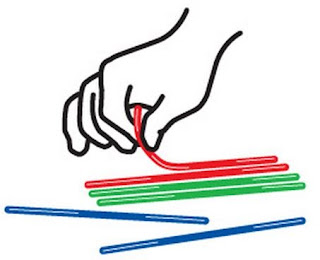Friday, June 28, 2013

Just DO MATH!

Do MATH!  This summer I keep on thinking how I want my students to do math.  Actually DO MATH.  Meaning I want them to use their hands to explore and accomplish MATH.  I want them to measure with a ruler or tape measure.  I want them to use a protractor.  I want them to investigate with some actual data that they have collected.  I want them to construct.  I want them to cut and paste.  I want them to use pipe cleaners.  I want them to use Wikistix to create a graph.  They should be actually constructing something and numbers should be going through their heads.

Let me give you two phrases and you choose the one you like the best.
1.  Regurgitate math
2.  Experience math
Exactly.  I would choose 2 also.  But what do we make our students choose time and time again? Yep, #1.

Here is the origin of this idea Do MATH.  Last spring I had my Precalculus students use a 30-60-90 triangle in a video.  Basically they had to construct a 30-60-90 triangle to help them explain some other concept that we were covering. Interestingly, the students didn't have any trouble with the concept we were covering but they did have major questions of "How do I make a 30-60-90 triangle?"  This was just mind-boggling to me.  These are the students who could tell you the exact distances of each side of a 30-60-90 triangle for just about any length of any side.  Now they wanted to know how to construct it?  Well, I had to rethink my own teaching.  Have I ever taught them how to make a 30-60-90 triangle?  Have they ever had the practical end of the trigonometry?  So I realized that my students are not at fault.  I AM AT FAULT!!!!!  I need to have my students DO MATH.  I will make time because I don't want my students to walk out of my class without some practical knowledge of their math instead of just theory.WikiStix
Here are the ideas that I have been thinking about.  I have included the level for when this might be covered. You will notice there are a lot of Introduction to Algebra and Precalculus Ideas.  That is what I'm teaching now.
1.  Precalculus:  Construct a 30-60-90 triangle with cardboard or some other material.  One side must be at least 10 inches.   Justify in two ways that you know this is exactly a 30-60-90 triangle.    Others:  Construct a 45-45-90 triangle.
2.  Introduction to Algebra:  Build a Balance Scale.  Use any materials needed for the project.  You will be using pennies as your "weights".  You will be required to show the penny weight of a few different objects.  Notes:  I see this as an important link in how to solve equations.  This scale could be used when solving different equations.
3.  Introduction to Algebra:  Create a number line on the floor with blue masking tape.  You should mark out the numbers from -5 to 5.  Each number should have 10.5 inches between them.  You will be using these to add and subtract integers by standing up and moving to a problem.
4.  Precalculus:  Make a WikiStix parabola.  You must make it to be at least 15 inches in one direction.  You must be ready to justify why you know this is a parabola.   You must give the equation for the parabola.
Other ideas:  Create a sine graph. Create a  tangent graph.
5.  Introduction to Algebra:  Create a circle and  rectangle that have exactly 50 square inches of area inside.
6.  Precalculus:  Create a WikiStix triangle that shows how the ambiguous case is solved.